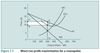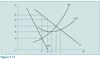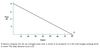Module 7 - Organisation of Industries Flashcards Preview

Economics > Module 7 - Organisation of Industries > Flashcards

Flashcards in Module 7 - Organisation of Industries Deck (81):
1

1

2

3

4

5

5

6

6

7

8

9

10

10

11

11

12

12

12

13

14

14

15

16

17

Draw short run profit maximisation for a monopolist19

20

Assume the jumps were completely automated so that variable costs were zero what price per jump would result in a maximum profit and why? A. \$2.00 because demand is price elastic B. \$4.00 because marginal revenue is zero when output = 40 C. \$6.00 because demand is price inelastic D. The answer cannot be determined from the diagram21

At which price would a monopolist whose cost and revenue curves are those pictured in Figure 7.17 maximise profit? A. B. B. C. C. D. D. E.22

22

If the leisure company wishes to maximise daily revenue what price should it charge and how many jumps will it sell? A. \$2.00 and 80 jumps B. \$4.00 and 40 jumps C. \$6.00 and 30 jumps D. The answer cannot be determined from the diagram23

What is the largest output the monopolist in Figure 7.17 could produce without suffering losses? A. G. B. H. C. I. D. J.24

25

26

Explain why an ‘enforced’ competitive industry would not only have higher per unit costs but could also yield a lower total output than the monopoly.27

28

29

29

30

31

If the average total cost (ATC) of operating the jump were constant at all levels of output and equalled \$4.00 what would be the profit maximising output and price? A. 60 and \$2.00 B. 40 and \$4.00 C. 20 and \$6.00 D. The answer cannot be determined from the diagram and data31

32

If the demand curve were to shift to the left and average variable costs were to stabilise at \$4.00 per jump for all levels of output what would be the profit maximising price? A. \$5.00 B. \$6.00 C. \$7.00 D. The answer cannot be determined from the diagram and information given33

33

34

36

38

40

41

43

45

45

48

49

50

52

54

55

56

57

59

60

61

The monopolist’s average revenue curve A. is the line labelled MR. B. is the line labelled D. C. is the line labelled MC. D. does not appear on the Figure.62

62

63

64

65

67

69

71

72

Draw avgerage and marginal curve under monopoly...73

74

75

76

77

78

79

80

81